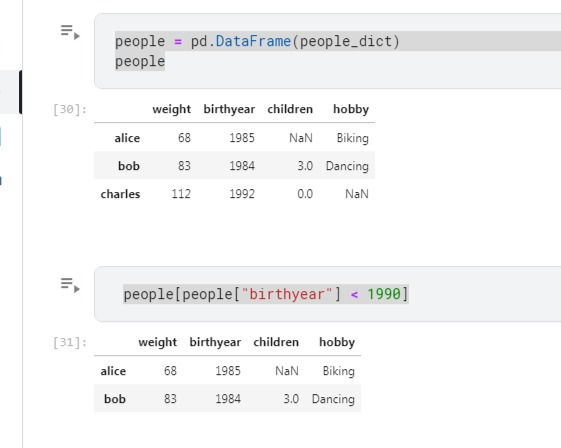top of pageSearch

# Begin With Pandas In 5minsPandas is an open-source library that is built on top of the NumPy library. It is a Python package that offers various data structures and operations for manipulating numerical data and time series.

It is mainly popular for importing and analyzing data much easier. Pandas is fast and it has high performance & productivity for users.

NumPy library which provides objects for multi-dimensional arrays, pandas will provide an in-memory 2d table object called Dataframe. so pandas will have many additional functionalities like plotting graphs, creating pivot tables.

# Creating a data frame using List: DataFrame can be created using a single list or a list of lists.

```# import pandas as pd
import pandas as pd

# list of strings
lst = ['python', 'java', 'oops']

# Calling DataFrame constructor on list
df = pd.DataFrame(lst)
print(df)```

```     0
0  python
1    java
2    oops```

Dataframe can be visualized as dictionaries of Series. Dataframe will be in the form of rows and columns, data

```people_dict = { "weight": pd.Series([68, 83, 112],index=["alice", "bob", "charles"]),
"birth year": pd.Series([1984, 1985, 1992],
index=["bob", "alice", "charles"], name="year"),
"children": pd.Series([0, 3], index=["charles", "bob"]),
"hobby": pd.Series(["Biking", "Dancing"], index=["alice", "bob"]),}```New columns and rows can be easily added to the data frame. In addition to the basic functionalities, the panda's data frame can be sorted by a particular column.

head(): returns the top 5 rows in the data frame object

tail(): returns the bottom 5 rows in the data frame

info(): prints the summary of the data frame

describe(): gives a nice overview of the main aggregated values over each columnSo it is a small walkthrough over the concept of Pandas, Every time when we start learning Pandas, there is a chance that you may get wandering off in the Pandas like index, functions, NumPy, etc., but we should understand the concept basics don’t let confusion to reach us.

Finally we clearly have to understand is that Pandas is a tool to visualize and get a deeper understanding of your data.

Here is the Link of my Kaggle Notebook to get started

References : https://www.geeksforgeeks.org/pandas-tutorial/